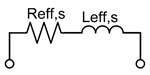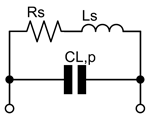inductance calculator, by Serge Stroobandt, ON4AARound wire coil with dimensions and its current-sheet approximation D mm Mean diameter of the air core coil, measured from wire centre to wire centre N Number of turns ℓ mm Lenght of the coil, measured from the connecting wires centre to centre d mm Wire or tubing diameter Plating material ρ nΩ·m Plating conductivity μr Plating permeability f MHz Design frequency INTERMEDIATE RESULTS: p mm Winding pitch Φ Proximity factor according to empirical Medhurst data[2,3] Deff mm Effective coil diameter according to Stroobandt (see below) Correction factors: kL Field non-uniformity correction factor according to Lundin[2,4] ks Round wire self-inductance correction factor according to Rosa[2,5,6] km Round wire mutual-inductance correction factor according to Grover and Knight[2,7] Wire: ℓwire, phys mm Physical wire length ℓwire, eff mm Effective wire length δi µm Skin depth at design frequency Sheath helix waveguide mode ψ ° Effective pitch angle β rad/m Axial propagation factor of n=0 sheath helix waveguide mode at design frequency[1,8] Zc Ω Characteristic impedance of n=0 sheath helix waveguide mode at design frequency Effective circuit equivalent at design frequencyLeff,s µH Effective series inductance at design frequency from Corum & Corum's sheath helix waveguide formula, corrected for field non-uniformity and round wire[1,2,4-7] Xeff,s Ω Effective series reactance of round wire coil at design frequency Reff,s Ω Effective series AC resistance of round wire coil at design frequency Qeff,ul Effective unloaded quality factor of round wire coil at design frequency Lumped circuit equivalent at design frequencyLs µH Frequency-independent series inductance from the current-sheet coil geometrical formula, corrected for field non-uniformity and round wire[2,4-7] XL,s Ω Series reactance of round wire coil RL,s Ω Series AC resistance of round wire coil at design frequency QL,ul Unloaded quality factor of round wire coil at design frequency CL,p pF Parallel stray capacitance at design frequency Self-resonant frequency: fres,L MHz λ/4 (parallel) self-resonant frequency of n=0 sheath helix mode[1,8]